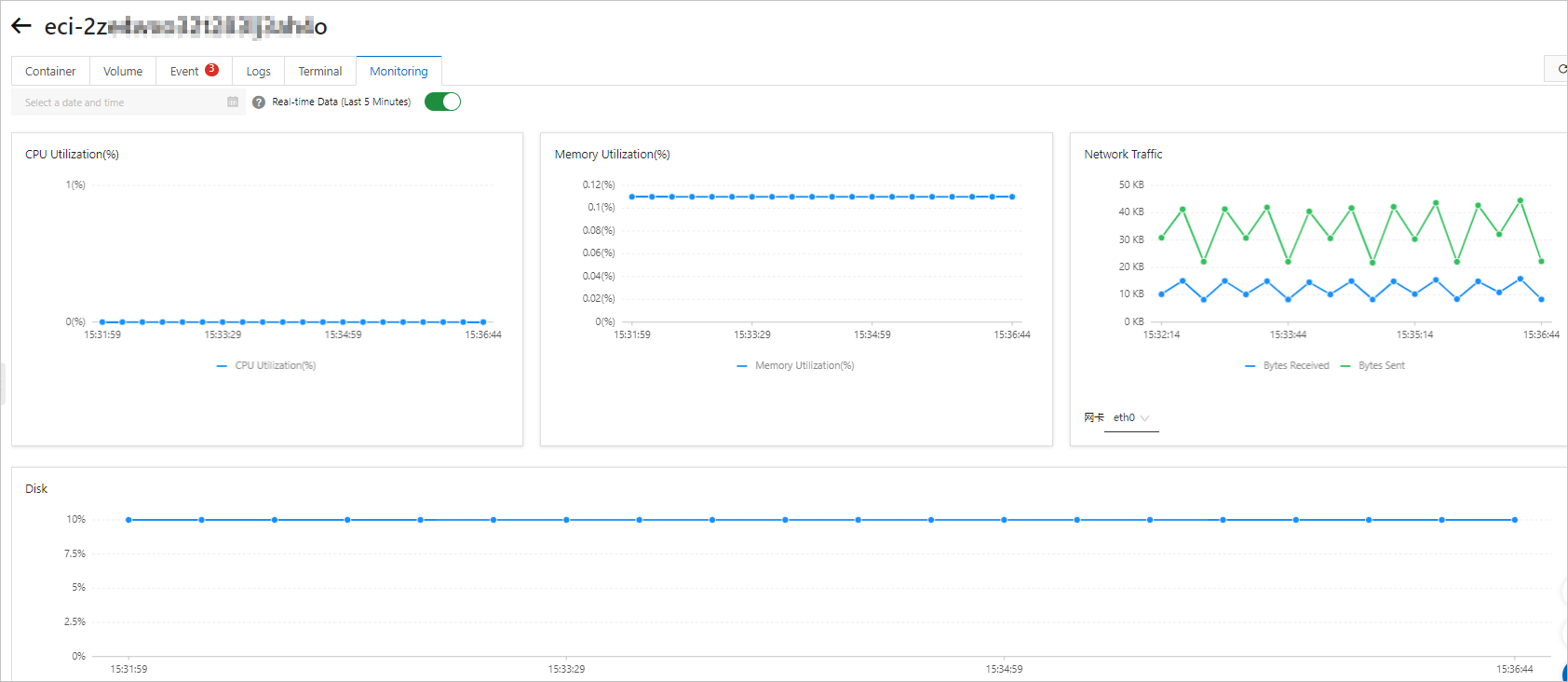Search
Document Center

# View the monitoring metrics of an elastic container instance

Last Updated: Oct 14, 2021

The monitoring data of elastic container instances are displayed in the Elastic Container Instance console. You can view monitoring data such as the metrics related to vCPUs, memory, and network resources. This topic describes the definitions of the monitoring metrics and how each metric is calculated.

## Metrics overview

Elastic Container Instance provides real-time monitoring data and historical data. The real-time data shows the metrics of the instance in the last 5 minutes, and the historical data shows the metrics of the instance in the past hour. You can configure monitoring for the following metrics:

• vCPU

This metric indicates the vCPU utilization. The figure shows the total monitoring data of multiple vCPUs. The upper limit is the number of vCPUs in the elastic container instance. For example, if the vCPU utilization is 150%, 1.5 vCPUs are used.

• Memory

This metric indicates the memory utilization of the elastic container instance. The upper limit is 100%.

• Network

This metric indicates the total number of bytes that are transmitted and received in the specified time window.

• Disk

This metric indicates the monitoring data of disk partitions and spaces:

• Monitoring data of disk partitions: includes data about system partitions and data partitions. Data partitions are partitions of disks that are mounted as data disks.

• Monitoring data of disk space: includes data about the total size of disk space, the size of used space, the size of remaining free space, and the disk space utilization.You can call the DescribeContainerGroupMetric or DescribeMultiContainerGroupMetric operation to query the monitoring data of one or more elastic container instances, and then use the monitoring data for secondary development and computing. When you query the monitoring data, the system returns the monitoring data of both the container group and the containers in the container group.

• The Records root node of the returned structure contains the monitoring data of the entire container group, including the data of the vCPUs, disks, memory, and network.

• The Containers child node of the returned structure contains the monitoring data of each container, including the data of the vCPUs and memory.

## Calculation methods for vCPUs metrics

When you call the DescribeContainerGroupMetric or DescribeMultiContainerGroupMetric operation, you can obtain the following raw data of vCPUs.

Parameter

Type

Example

Description

UsageNanoCores

Long

0

The vCPU usage in the sampling window. Unit for the sampling window: nanoseconds.

UsageCoreNanoSeconds

Long

70769883
The cumulative usage of vCPUs.

Long

0

The average load in the last 10 seconds.

Limit

Long

2000

The upper limit of vCPU usage. The calculation formula for this parameter: The number of vCPUs × 1000.

The vCPU-related metrics are calculated by using the following formulas:

• vCPU usage = UsageNanoCores/109

• vCPU utilization = UsageNanoCores/Limit/106

## Calculation methods for memory metrics

When you call DescribeContainerGroupMetric or DescribeMultiContainerGroupMetric, you can obtain the following raw data of the memory.

Parameter

Type

Example

Description

AvailableBytes

Long

4289445888

The size of the available memory. Unit: bytes.

UsageBytes

Long

11153408

The size of the used memory. Unit: bytes.

Cache

Long

7028736

The size of the cache. Unit: bytes.

WorkingSet

Long

5521408

The usage of the memory working set. Unit: bytes.

Long

1593344

The size of the resident memory set, which indicates the size of the physical memory that is actually used. Unit: bytes.

The memory-related metrics are calculated by using the following formula:

Memory utilization = WorkingSet/(WorkingSet + AvailableBytes)

## Calculation methods for network metrics

When you call DescribeContainerGroupMetric or DescribeMultiContainerGroupMetric, you can obtain the following raw data of the network.

Parameter

Type

Example

Description

TxBytes

Long

1381805699

The total number of bytes transmitted

RxBytes

Long

505001954

The total number of bytes received

TxErrors

Long

0

The total number of transmission errors

RxErrors

Long

0

The total number of receipt errors

TxPackets

Long

5158427

The total number of packets transmitted

RxPackets

Long

4800583

The total number of packets received

TxDrops

Long

0

The number of transmitted packets that fail to arrive at their destination

RxDrops

Long

0

The number of packets that fail to be received

Name

String

eth0

The name of the network interface controller (NIC)

The network-related metrics are calculated by using the following formulas:

• Bandwidth rate: number of bits transmitted per second. Unit: bit/s.

Bandwidth rate = (The total number of bytes transmitted at time B - The total number of bytes transmitted at time A)/Number of seconds between time A and time B × 8

• Throughput rate: number of packets transmitted per second. Unit: pps.

Throughput rate = (The total number of packets transmitted at time B - The total number of packets transmitted at time A)/Number of seconds between time A and time B